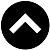-->

## MATHEMATICS FORM 3 SYLLABUS

1. Relations
1. Relations
1. Find relations between two sets
2. Find relations between members in a set
3. Demonstrate relations pictorially
2. Graph of a Relation
1. Draw a graph of a relation represented by a linear inequality
3. Domain and Range of a Relation
1. State the domain of relation
2. State the range of a relation
4. Inverse of a Relation
1. Explain the Inverse of a relation pictorially
2. Find inverse of a relation
3. Draw a graph of the inverse of a relation
2. Functions
1. Representation of a Function
1. Explain the concept of a functions pictorially
2. Identify functions
2. Domain and Range of a Function
1. State the domain of a function
2. State the range of function
3. Graphic Function
1. Draw graphs of functions
4. Inverse of a Function
1. Explain the inverse of a function
2. Show the inverse of a function pictorially
3. Find the inverse of a function
4. Draw a graph of the inverse of a function
5. State the domain and range of inverse of functions
3. Statistics
1. Mean
1. Calculate the mean from a set of data, frequency distribution tables and histogram
2. Interpret the mean obtained from a set data, frequency distribution tables and histogram
2. Medium
1. Explain the concept of median
2. Calculate the medium from a set of data
3. Find the median using frequency distribution tables and cumulative curve
4. Interpret the median obtained from the data
3. Mode
1. Explain the concept of mode
2. Calculate the mode
3. Find the mode using frequency distribution and a histogram
4. Interpret the mode obtained from the data
4. Rates And Variations
1. Rates
1. Relate rates of quantities of different kinds
2. Relate quantities of the same kind
3. Convert Tanzanian currency into other currencies
2. Variations
1. Explain the concept of direct variation
2. Solve problems on direct variations
3. Draw graphs of direct variation
4. Explain the concept of inverse variation
5. Solve problems on inverse variations
6. Draw graphs relating inverse variations
7. Use joint variation in solving problems
5. Sequence And Series
1. Sequences
1. Explain the concept of sequence
2. Identify an arithmetic progression (AP) and geometric progression (GP)
3. Find the general term of an AP
4. Find the general term of GP
2. Series
1. Derive the formula for a sum of an arithmetic progression
2. Calculate the arithmetic mean
3. Derive the formula for the sum of a geometric progression
4. Calculate the geometric mean
3. Compound Interest
1. Calculate compound interest using formula
6. Circles
1. Definition of Terms
1. Define circle, chord, radius, diameter, circumference, arc, sector, centre and segment of a circle
2. Central Angle
1. Derive the formula for the length of an arc
2. Calculate the central angle
3. Explain the concept of radian measure
4. Convert radians to degree and vice versa
3. Angles Properties
1. Prove circle theorems of inscribed angles
2. Apply the circle theorems in solving related problems
4. Chord Properties of a Circle
1. Identify chord properties of a circle
2. Prove the theorem on the perpendicular bisector to a chord.
3. Prove the theorem on parallel chords
4. Apply the theorems on chords in solving related problems
5. Tangent Properties
1. Describe a tangent to a circle
2. Identify tangent properties of a circle
3. Prove tangent theorems
4. Apply theorems relating to tangent to a circle in solving problems
7. The Earth As A Sphere
1. Features and Location of Places
1. Describe the equator, great circle, small circles, meridian, latitudes and longitudes
2. Locate a place on the Earth’s surface
2. Distances along Great Circles
1. Calculate distances along great circles
3. Distances along Small Circles
1. Calculate distance along small circles
8. Accounts
1. Double Entry
1. Explain the meaning of double entry
2. Explain different types of ledger
3. Construct a ledger
4. Post entries in the ledger
5. Close the simple accounts
2. Trial Balance
1. Explain the concept of trial balance
2. Construct trial balance
3. Post debit balances and credit balances
4. Check the balances